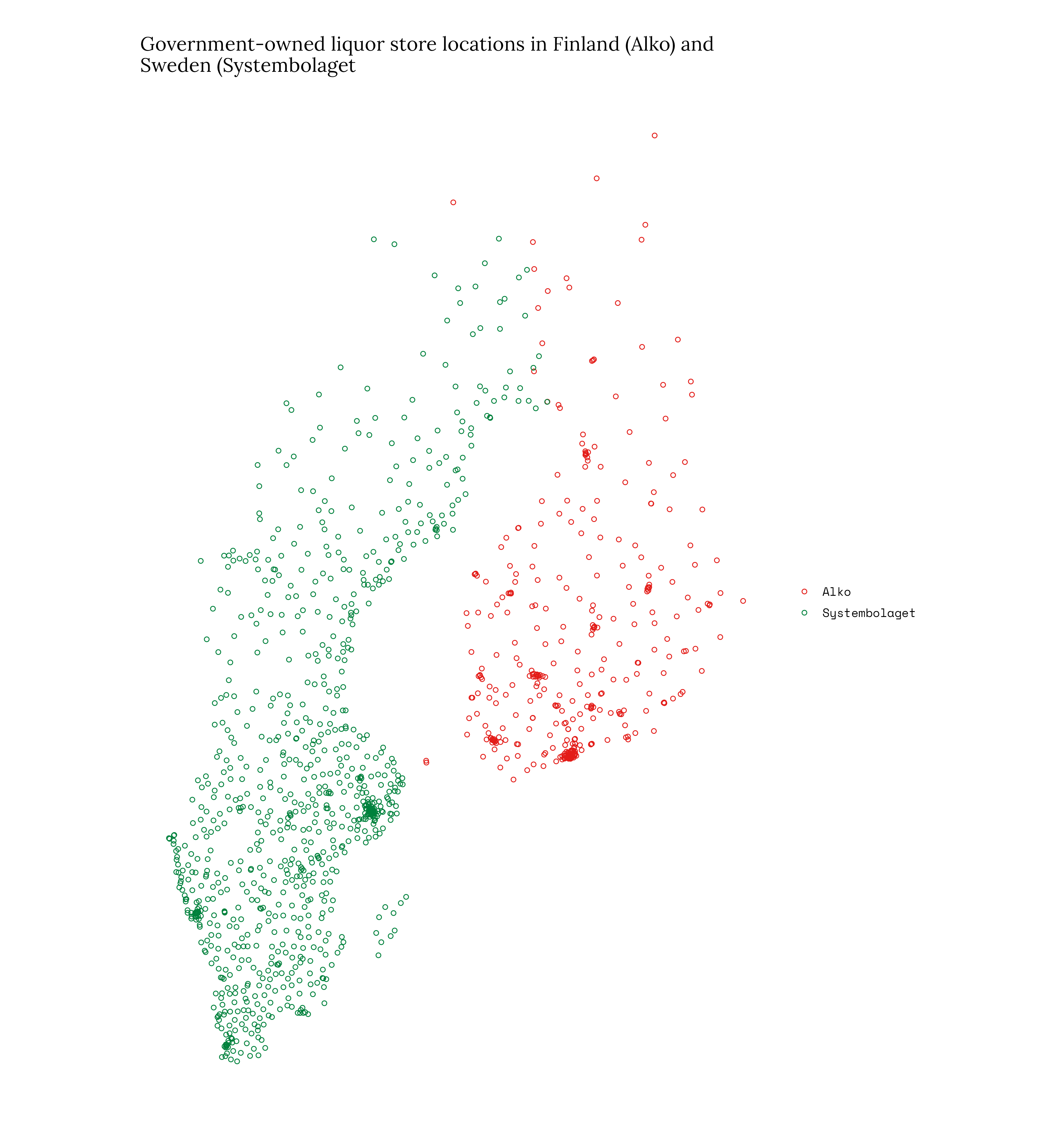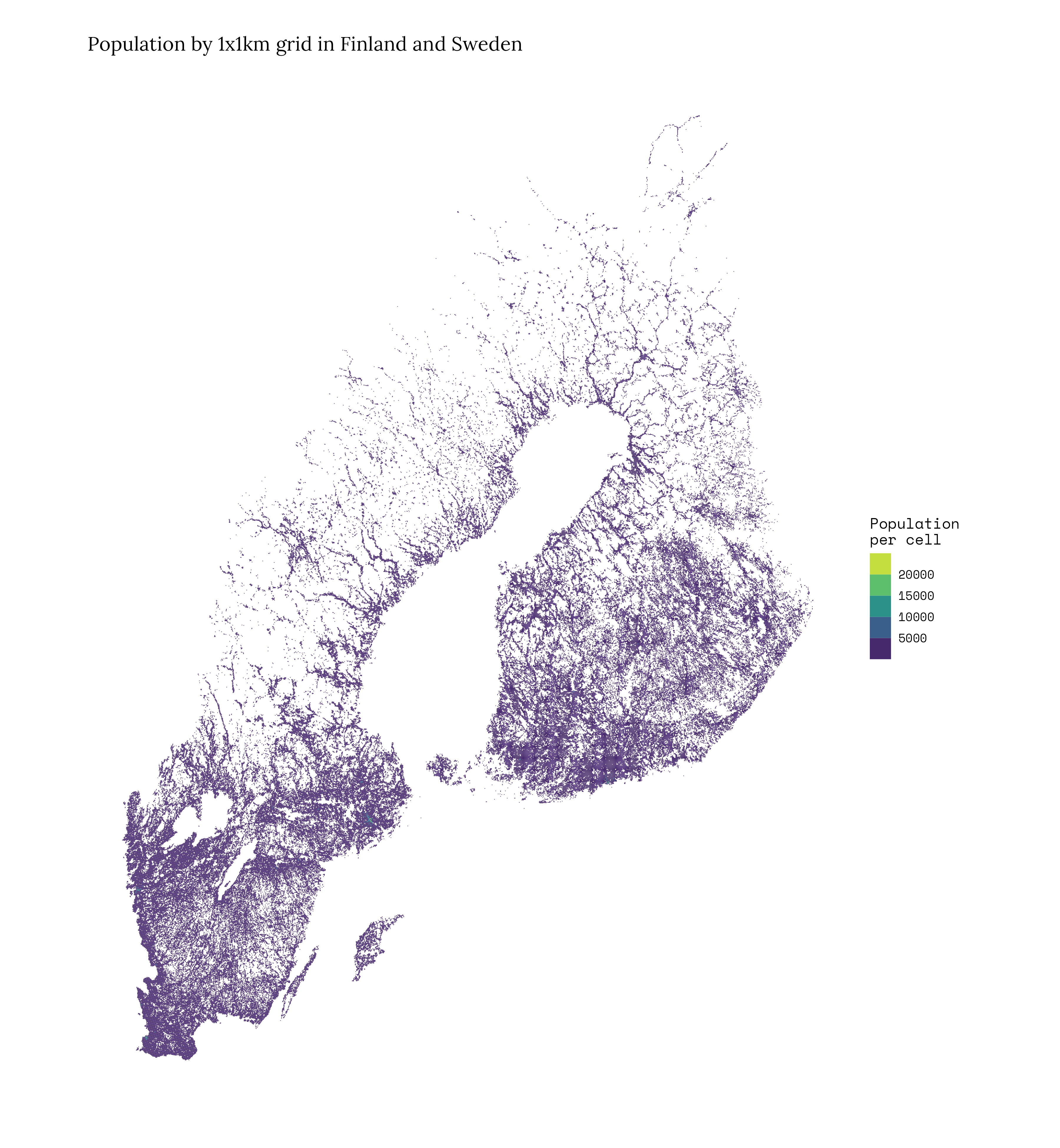# Who lives closest to liquor store, Finns or Swedes?

data
alcohol
spatial
api
web scraping
docker

This is not a study, but another technical demo on how to use data from websites and proper spatial data sources in R programming language. Vague research question that I am looking to answer is “In which county, Sweden or Finland, people have better access to state liquor stores”.

In case you are only interested in the answer, by no surprise, the state supply of also alcohol is better taken care in Sweden. The mean distance to nearest liquor store by road in Sweden is 4.50km, whereas in Finland you have to ride your bicycle more than a kilometer longer. Mean distance in Finland is 5.95km.

## Introduction

These two countries are chosen because they both have a state monopoly in alcohol retail, Alko in Finland and Systembolaget in Sweden.

Also, you there is population grid data openly available from both countries, at Statistics Finland and at Statistics Sweden.

## Liquor store location data

To get the locations of liquor stores you need to apply http `GET`queries in the case of Systembolaget and do shady `web scraping` in the case of Alko in Finland. Point coordinates are in `WGS84` coordinate reference system by default that you need to transform into `ESPG:3067` which isthe projected coordinate system for Finland that I use here.

``````library(httr2)
library(dplyr)
library(jsonlite)
library(ggplot2)
library(sf)

req <- request("https://www.systembolaget.se/api/gateway/sitesearch/site/")
req <- req %>% req_headers("baseURL" = "https://api-systembolaget.azure-api.net/sb-api-ecommerce/v1")

# req_dry_run(req)
resp <- req_perform(req)
systembolaget_list <- jsonlite::fromJSON(resp_body_string(resp))

systembolaget_df <- bind_cols(
systembolaget_list\$siteSearchResults %>%
select(siteId,alias,city),
systembolaget_list\$siteSearchResults\$position
) %>%
filter(!is.na(longitude),
latitude >= 10)
systembolaget_sf <- sf::st_as_sf(systembolaget_df, coords = c("longitude","latitude"), crs = "EPSG:4326") %>%
st_transform(crs = 3067) %>%
mutate(store_name = as.character(siteId))

library(rvest)

nayta_lisatiedot <- html %>%
html_elements(".show-info") %>%
html_attrs()

lista <- list()
for (i in seq(nayta_lisatiedot)){
print(paste0(i, "/", length(nayta_lisatiedot)))
sijainti_lst <- html_lisatiedot %>%
html_elements("meta") %>%
html_attrs()

for (ii in seq(sijainti_lst)){
if (any(grepl("property", names(sijainti_lst[[ii]])))){
# Lets pick the name
if (sijainti_lst[[ii]][] == "og:title"){
name <- sijainti_lst[[ii]][]
}
# lets pick the location
if (sijainti_lst[[ii]][] == "og:image"){
loc <- strsplit(gsub("^.+center=|&size.+\$", "", sijainti_lst[[ii]][]), split = ",") |> unlist()
}
# lets pick the location
if (sijainti_lst[[ii]][] == "og:image"){
loc <- strsplit(gsub("^.+center=|&size.+\$", "", sijainti_lst[[ii]][]), split = ",") |> unlist()
}
} else next()
}
lista[[i]] <- tibble(name = name, lat = loc, lng = loc)
rm(name)
rm(loc)
}
alko_sf <- do.call("bind_rows", lista) %>%
sf::st_as_sf(coords = c("lng","lat"), crs = "EPSG:4326") %>%
mutate(type = ifelse(grepl("Noutopiste", name), "take-away", "store"))

alko_sf_store <- alko_sf %>%
filter(type == "store") %>%
mutate(store_name = name) %>%
st_transform(crs = 3067)

# Plot the store locations
stores_swe_fin <- rbind(alko_sf_store %>% select(store_name),
systembolaget_sf %>% select(store_name)) %>%
mutate(bolag = ifelse(grepl("alko|Alko", store_name), "Alko", "Systembolaget"))

alko_swe_fin_plot <- ggplot() +
geom_sf(data = stores_swe_fin, aes(color = bolag), shape = 21) +
scale_color_manual(values = c("#e31c18", "#00813c")) +
labs(title = "Government-owned liquor store locations in Finland (Alko) and \nSweden (Systembolaget",
color = NULL)
alko_swe_fin_plot``````## Population grid data

For Finnish data you can use functions from geofi-package. Statistics Sweden disseminates spatial data in `geopackage`-format. Just download the zipped file, unzipt and read to R.

``````# Finland
grid_finland <- geofi::get_population_grid(resolution = 1) %>%
mutate(grid_id = id_nro)

# Sweden
tmpfly <- tempfile()
tmpdir <- tempdir()
unzip(zipfile = tmpfly, exdir = tmpdir)
filename <- grep("gpkg", fs::dir_ls(tmpdir), value = TRUE)
grid_sweden_espg3067 <- sf::st_read(filename, crs = 3006) %>%
sf::st_transform(crs = 3067) %>%
mutate(vaesto = Pop,
grid_id = Ruta
)
# grid
grid_swe_fin <- rbind(grid_finland %>% select(vaesto),
grid_sweden_espg3067 %>% select(vaesto))

grid_swe_fin_plot <- ggplot() +
geom_sf(data = grid_swe_fin, aes(fill = vaesto), color = NA) + scale_fill_viridis_b() +
labs(title = "Population by 1x1km grid in Finland and Sweden", fill = "Population \nper cell")
grid_swe_fin_plot``````## Distances between grid cells and stores

Computing distance between to points or to sets of points is straightforward and quick in R. We do this because later on, we only want to compute routes to nearest store to save time and electricity.

``````library(osrm)
library(dplyr)
library(sf)
library(geofi)

# Finland
stores_fin <- alko_sf_store
# grid data into centroids (points)
population_grid_fin <- grid_finland %>%
st_centroid()

# distances as crow flies
dists <- st_distance(x = population_grid_fin, y = stores_fin)

dist_list <- list()
for (i in 1:nrow(dists)){
if (i%%1000 == 0) print(paste0(i,"/",nrow(dists)))
dist_list[[i]] <- tibble(store_name = stores_fin\$store_name,
distance = dists[i,]) %>%
mutate(grid_id = population_grid_fin\$grid_id[i])
}
dist_df_fin <- as.data.frame(data.table::rbindlist(dist_list))

dist_df_fin_shortest <- dist_df_fin %>%
mutate(distance = as.numeric(distance)) %>%
group_by(grid_id) %>%
filter(distance == min(distance)) %>%
ungroup() %>%
left_join(st_drop_geometry(population_grid_fin))

# compute the mean distance per person
tot_dist <- dist_df_fin_shortest %>%
summarise(total_distance = distance/1000 * vaesto) %>%
summarise(total_distance = sum(total_distance))
tot_pop <- dist_df_fin_shortest %>%
summarise(total_population = sum(vaesto))
mean_dist_fin <- tot_dist/tot_pop
# 4.178743``````
``````# Sweden
stores_swe <- systembolaget_sf
# grid data into centroids (points)
population_grid_swe <- grid_sweden_espg3067 %>%
st_centroid()

# distances as crow flies
dists <- st_distance(x = population_grid_swe, y = stores_swe)

dist_list <- list()
for (i in 1:nrow(dists)){
if (i%%1000 == 0) print(paste0(i,"/",nrow(dists)))
dist_list[[i]] <- tibble(store_name = stores_swe\$store_name,
distance = dists[i,]) %>%
mutate(grid_id = population_grid_swe\$grid_id[i])
}
dist_df_swe <- as.data.frame(data.table::rbindlist(dist_list))

dist_df_shortest_swe <- dist_df_swe %>%
mutate(distance = as.numeric(distance)) %>%
group_by(grid_id) %>%
filter(distance == min(distance)) %>%
ungroup() %>%
left_join(st_drop_geometry(population_grid_swe))

# compute the mean distance per person
tot_dist <- dist_df_shortest_swe %>%
summarise(total_distance = distance/1000 * vaesto) %>%
summarise(total_distance = sum(total_distance))
tot_pop <- dist_df_shortest_swe %>%
summarise(total_population = sum(vaesto))
mean_dist_swe <- tot_dist/tot_pop
# 2.932753``````

As we have now calculated the as-crow-flies distances, we can conclude that that Swedes only have to fly, on average, 2.93 km to get their bottle of Absolut, while Finns need to take a lot longer flight of 4.18 km for their Koskenkorva.

But as a drunk people are not allowed to fly, we need to calculate the distances by road that they can walk. Here we assume, that the nearest alcohol store by flying would be the nearest also by road, we only do routing for these trips. (This is not the case for instance in the Archipelago!)

## Routing through road network using Open Source Routing Machine (OSRM)

For routing we could use a commercial routing APIs like Google or Yahoo, but let’s rather create our own for the sake of privacy, speed and costs. Learn Open Source Routing Machine if you haven’t already.

### Configure OSRM Docker containers

For the routing exercise you need to have docker installed and some file download utility, I use `wget` here. The docker commands below are meant to run in os terminal, not in R!

First prepare the OSRM containers for both countries.

``````# Download the background image
docker pull osrm/osrm-backend
# Tag different version for Finland and Sweden
docker tag osrm/osrm-backend osrm-backend:finland
docker tag osrm/osrm-backend osrm-backend:sweden

## Finland

# extract
docker run -t -v "\${PWD}:/data" osrm-backend:finland osrm-extract -p /opt/car.lua /data/finland-latest.osm.pbf
# partition
docker run -t -v "\${PWD}:/data" osrm-backend:finland osrm-partition /data/finland-latest.osrm
# customize
docker run -t -v "\${PWD}:/data" osrm-backend:finland osrm-customize /data/finland-latest.osrm

## Sweden

# extract
docker run -t -v "\${PWD}:/data" osrm-backend:sweden osrm-extract -p /opt/car.lua /data/sweden-latest.osm.pbf
# partition
docker run -t -v "\${PWD}:/data" osrm-backend:sweden osrm-partition /data/sweden-latest.osrm
# customize
docker run -t -v "\${PWD}:/data" osrm-backend:sweden osrm-customize /data/sweden-latest.osrm``````

The start the two containers before you fire up the routing in the R code below.

``````## Launch Finland
docker run -t -i -p 5000:5000 -v "\${PWD}:/data" osrm-backend:finland osrm-routed --algorithm mld /data/finland-latest.osrm
## Launch Sweden
docker run -t -i -p 5001:5000 -v "\${PWD}:/data" osrm-backend:sweden osrm-routed --algorithm mld /data/sweden-latest.osrm``````

### Run the routing

Once the containers are waiting for requests, let’s run the R code below to get the distances and routes along the roads.

``````# swe
destination <- stores_swe %>%
right_join(dist_df_shortest_swe)
origin <- population_grid_swe %>%
right_join(dist_df_shortest_swe)

options(osrm.server = "http://127.0.0.1:5001/")

origins <- unique(origin\$Ruta)
list1 <- list()
for (i in seq(origins)){
if (i%%100 == 0) print(paste0(i,"/",length(origins)))

tmp_res <- osrmRoute(src = origin[origin\$Ruta %in% origins[i],],
dst = destination[destination\$grid_id %in% origins[i],],
overview = "simplified"
)
list1[[i]] <- tmp_res %>%
mutate(
grid_id = origins[i],
store_name = stores_swe\$name[i]
)
}
df_routes_swe <- as.data.frame(data.table::rbindlist(list1))
lines_swe <- df_routes_swe\$geometry
lines_swe_sf <- sf::st_as_sf(lines_swe)
lines_swe_sf <- cbind(lines_swe_sf,df_routes_swe %>% select(-geometry))

# fin
destination <- stores_fin %>%
right_join(dist_df_shortest_fin)
origin <- population_grid_fin %>%
right_join(dist_df_shortest_fin)

options(osrm.server = "http://127.0.0.1:5000/")
origins <- unique(origin\$grid_id)
list1 <- list()
for (i in 11567:length(origins)){
# for (i in seq(origins)){
if (i%%100 == 0) print(paste0(i,"/",length(origins)))

tmp_res <- osrmRoute(src = origin[origin\$grid_id %in% origins[i],],
dst = destination[destination\$grid_id %in% origins[i],],
overview = "simplified"
)
list1[[i]] <- tmp_res %>%
mutate(
grid_id = origins[i],
store_name = stores_fin\$name[i]                           )
}

df_routes_fin <- as.data.frame(data.table::rbindlist(list1))
lines_fin <- df_routes_fin\$geometry
lines_fin_sf <- sf::st_as_sf(lines_fin)
lines_fin_sf <- cbind(lines_fin_sf,df_routes_fin %>% select(-geometry))``````

It took some time, but we are ready now. What are the mean distances along roads then?

``````# For Finland

summarise(total_distance = distance * vaesto) %>%
summarise(total_distance = sum(total_distance))
summarise(total_population = sum(vaesto))
# 5.945485

# For Sweden

summarise(total_distance = distance * vaesto) %>%
summarise(total_distance = sum(total_distance))
summarise(total_population = sum(vaesto))
# 4.499895``````

## Results

As stated at the beginning, Swedes live slightly closer to liquor stores, on average, than Finns. 4.499895 kilometers vs. 5.945485 kilometers.

Let’s create the final plot, with the shortest routes and the liquor stores combined with the following code.

``````lines_both <- rbind(lines_swe_sf %>% select(distance),
lines_fin_sf %>% select(distance))

routes_both <- ggplot(lines_both #%>% sample_n(size = 100000)
) +
geom_sf(alpha = .01) +
geom_sf(data = stores_swe_fin, aes(color = bolag), size = .1) +
scale_color_manual(values = c("#e31c18", "#00813c")) +
labs(title = "Shortest routes to nearest liquor store from each 1x1km population cell \nin Finland and Sweden",
color = NULL)
routes_both``````Click to a larger version

## Extra: Stockholm and Helsinki

Lets also plot similar maps of the capital cities, ie. 20 km boundaries around the parliament houses. Coordinates are easy to find from Wikipedia articles: Riksdag and Parliament of Finland.

``````parliament_fin <- tibble(lon = 24.933333, lat = 60.1725) %>%
sf::st_as_sf(coords = c("lon","lat"), crs = 4326) %>%
st_transform(3067)
parliament_swe <- tibble(lon = 18.0675, lat = 59.3275) %>%
sf::st_as_sf(coords = c("lon","lat"), crs = 4326) %>%
st_transform(3067)

parliament_fin_buf <- st_buffer(x = parliament_fin, dist = 20000)
parliament_swe_buf <- st_buffer(x = parliament_swe, dist = 20000)

p1 <- ggplot() +
geom_sf(data = grid_finland %>% st_intersection(parliament_fin_buf), color = "white", fill = alpha("grey", 1/3)) +
geom_sf(data = lines_fin_sf %>% st_intersection(parliament_fin_buf), alpha = .6) +
geom_sf(data = alko_sf_store %>% st_intersection(parliament_fin_buf), color = "#e31c18", size = .8) +
geom_sf(data = parliament_fin, color = "blue") +
geom_sf(data = parliament_fin_buf, color = alpha("grey", 1/6), fill = NA) +
labs(title = "Helsinki")

p2 <- ggplot() +
geom_sf(data = grid_sweden_espg3067 %>% st_intersection(parliament_swe_buf), color = "white",  alpha("grey", 1/3)) +
geom_sf(data = lines_swe_sf %>% st_intersection(parliament_swe_buf), alpha = .6) +
geom_sf(data = systembolaget_sf %>% st_intersection(parliament_swe_buf), color = "#00813c", size = .8) +
geom_sf(data = parliament_swe, color = "blue") +
geom_sf(data = parliament_swe_buf, color = alpha("grey", 1/6), fill = NA) +
labs(title = "Stockholm")

p3 <- patchwork::wrap_plots(list(p1,p2), widths = c(1,1)) + patchwork::plot_annotation(title = "Distances to nearest liquor store from each 1x1km population cell within 20 km radius from Parliament house,  in Helsinki and Stockholm")``````CC BY 4.0

## Citation

BibTeX citation:
``````@online{kainu2023,
author = {Kainu, Markus},
title = {Who Lives Closest to Liquor Store, {Finns} or {Swedes?}},
date = {2023-01-17},
url = {https://markuskainu.fi/posts/2023-01-18-alko-systembolaget},
langid = {en}
}
``````